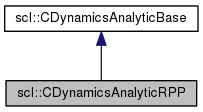SCL  1.0 Standard Control Library : Control, dynamics, physics, and simulation
scl::CDynamicsAnalyticRPP Class Reference

#include <CDynamicsAnalyticRPP.hpp>

Inheritance diagram for scl::CDynamicsAnalyticRPP:[legend]
Collaboration diagram for scl::CDynamicsAnalyticRPP:[legend]

Public Member Functions

virtual sBool computeTransformationMatrix (const Eigen::VectorXd &arg_q, sInt arg_link_id, sInt arg_ancestor_link_id, Eigen::Affine3d &arg_T)

virtual sBool computeJacobian (const Eigen::VectorXd &arg_q, sInt arg_link_id, const Eigen::VectorXd &arg_pos_local, Eigen::MatrixXd &arg_J)

virtual sBool computeGCModel (const Eigen::VectorXd &arg_q, SGcModel &arg_gc_model)

void computeTOrg_0 (const Eigen::VectorXd &arg_q, Eigen::Affine3d &arg_T)

void computeT0_1 (const Eigen::VectorXd &arg_q, Eigen::Affine3d &arg_T)

void computeT1_2 (const Eigen::VectorXd &arg_q, Eigen::Affine3d &arg_T)

bool computeJcom (const Eigen::VectorXd &arg_q, unsigned int arg_link_id, Eigen::MatrixXd &arg_J)

bool computeMgc (const Eigen::VectorXd &arg_q, Eigen::MatrixXd &arg_Mgc)

CDynamicsAnalyticRPP ()

virtual ~CDynamicsAnalyticRPP ()

virtual sBool init (const SRobotParsed &arg_robot_data)

virtual sBool hasBeenInit ()

Protected Attributes

Eigen::Vector3d pos_root_in_global_org_

sBool has_been_init_

Detailed Description

Implements analytical dynamics for the RPP bot.

For the details of the math used here, please see: Mathematica : doc/MathTutorial_01_RPPBot.nb

Constructor & Destructor Documentation

 scl::CDynamicsAnalyticRPP::CDynamicsAnalyticRPP ( )
inline

Default constructor sets the initialization state to false

 virtual scl::CDynamicsAnalyticRPP::~CDynamicsAnalyticRPP ( )
inlinevirtual

Default destructor does nothing

Member Function Documentation

 virtual sBool scl::CDynamicsAnalyticRPP::computeGCModel ( const Eigen::VectorXd & arg_q, SGcModel & arg_gc_model )
inlinevirtual

Calculates the Jacobian for the robot to which this dynamics object is assigned.

Uses id based link lookup. The dynamics implementation should support this (maintain a map or something).

Parameters
 arg_q The generalized coordinates arg_gc_model All individual dynamics matrices will be saved here.

Implements scl::CDynamicsAnalyticBase.

 virtual sBool scl::CDynamicsAnalyticRPP::computeJacobian ( const Eigen::VectorXd & arg_q, sInt arg_link_id, const Eigen::VectorXd & arg_pos_local, Eigen::MatrixXd & arg_J )
inlinevirtual

Calculates the Jacobian for the robot to which this dynamics object is assigned.

Uses id based link lookup. The dynamics implementation should support this (maintain a map or something).

Parameters
 arg_q The generalized coordinates arg_link_id The link at which the Jacobian is to be calculated arg_pos_local The offset from the link's frame (in local coordinates). arg_J The Jacobian will be saved here.

Implements scl::CDynamicsAnalyticBase.

 bool scl::CDynamicsAnalyticRPP::computeJcom ( const Eigen::VectorXd & arg_q, unsigned int arg_link_id, Eigen::MatrixXd & arg_J )

Compute the Jacobian that maps center of mass velocities in origin (global) coordinates to generalized velocities

 bool scl::CDynamicsAnalyticRPP::computeMgc ( const Eigen::VectorXd & arg_q, Eigen::MatrixXd & arg_Mgc )

Compute the generalized inertia matrix

Uses following inertial properties. Subscript[m, 0] = 10; Subscript[Ixx, 0] = 5; Subscript[Iyy, 0] = 5; Subscript[Izz, 0] = 2; Subscript[Ixy, 0] = 0; Subscript[Ixz, 0] = 0; Subscript[Iyz, 0] = 0; Subscript[m, 1] = 5; Subscript[Ixx, 1] = 3; Subscript[Iyy, 1] = 3; Subscript[Izz, 1] = 1; Subscript[Ixy, 1] = 0; Subscript[Ixz, 1] = 0; Subscript[Iyz, 1] = 0; Subscript[m, 2] = 3; Subscript[Ixx, 2] = 2; Subscript[Iyy, 2] = 2; Subscript[Izz, 2] = 0.5; Subscript[Ixy, 2] = 0; Subscript[Ixz, 2] = 0; Subscript[Iyz, 2] = 0;

Uses following com positions: Subscript[comPos, 0] = (0,0,-0.5) Subscript[comPos, 1] = (0,-.3625,0) Subscript[comPos, 2] = (0 0 .325)

 void scl::CDynamicsAnalyticRPP::computeTOrg_0 ( const Eigen::VectorXd & arg_q, Eigen::Affine3d & arg_T )

NOTE : The affine offset includes the offset of the global origin to the robot's origin. This is hard wired for now.

 sBool scl::CDynamicsAnalyticRPP::computeTransformationMatrix ( const Eigen::VectorXd & arg_q, sInt arg_link_id, sInt arg_ancestor_link_id, Eigen::Affine3d & arg_T )
virtual

Calculates the Transformation Matrix for the robot to which this dynamics object is assigned.

The Transformation Matrix is specified by a link and an offset (in task space dimensions)from that link and is given by:

Uses id based link lookup. The dynamics implementation should support this (maintain a map or something).

Parameters
 arg_q The generalized coordinates arg_link_id The link at which the transformation matrix is to be calculated arg_ancestor_link_id The link up to which the transformation matrix is to be calculated arg_T The transformation matrix will be saved here.

Implements scl::CDynamicsAnalyticBase.

inlinevirtual

Gives an id for a link name.

Useful because:

1. Allows storing any dynamic-engine specific objects in the controller.
2. Avoids using inefficient repeated string based lookup (usually with maps)

Implements scl::CDynamicsAnalyticBase.

 virtual sBool scl::CDynamicsAnalyticRPP::hasBeenInit ( )
inlinevirtual

Initialization state

Reimplemented from scl::CDynamicsAnalyticBase.

 sBool scl::CDynamicsAnalyticRPP::init ( const SRobotParsed & arg_robot_data )
virtual

The only state here is the position of the robot's ground wrt. the global origin. There is nothing to initialize since the entire dynamics engine is hard-coded for a robot and is otherwise stateless.

Returns, true : success false : failure

Implements scl::CDynamicsAnalyticBase.

Member Data Documentation

 sBool scl::CDynamicsAnalyticBase::has_been_init_
protectedinherited

True if the dynamics object has been initialized for a given robot

The documentation for this class was generated from the following files:
• /home/samir/Code/control/scl.git/src/scl/dynamics/analytic/CDynamicsAnalyticRPP.hpp
• /home/samir/Code/control/scl.git/src/scl/dynamics/analytic/CDynamicsAnalyticRPP.cpp